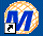# B8. Experimental Determination of Kinetic Parameters

## How can $$V_m$$ and $$K_m$$ be determined from experimental data?

From initial rate data: The most common way is to determine initial rates, $$v_0$$, from experimental values of $$P$$ or $$S$$ as a function of time. Hyperbolic graphs of $$v_0$$ vs. $$[S]$$ can be fit or transformed as we explored with the different mathematical transformations of the hyperbolic binding equation to determine $$K_d$$. These included:

• nonlinear hyperbolic fit
• double reciprocal plot
• Scatchard plot

The double-reciprocal plot is commonly used to analyze initial velocity vs. substrate concentration data. When used for such purposes, the graphs are referred to as Lineweaver-Burk plots, where plots of 1/v vs. 1/S are straight lines with slope $$m = \frac{KM}{V_{max}}$$, and y-intercept $$b = \frac{1}{V_{max}}$$. These plots can not be analyzed using linear regression, however, since that method assumes constant error in the y axis (in this case $$\frac{1}{v}$$) data. A weighted linear regression or even better, a nonlinear fit to a hyperbolic equation should be used. The Mathcad template below shows such a nonlinear fit. In the laboratory, we will use a series of programs developed by W. W. Cleland specifically designed to analyze initial rate data of enzyme catalyzed reactions. A rearrangement of the corresponding Scatchard equations in the Eadie-Hofstee plot is also commonly used.Mathcad 8 - Nonlinear Hyperbolic Fit. Vm and Km.

## Common Error in Biochemistry Textbooks

From integrated rate equations: $$K_M$$ and $$V_m$$ can be extracted from progress curves of $$A$$ or $$P$$ as a function of $$t$$ at one single $$A_0$$ concentration by deriving an integrated rate equation for $$A$$ or $$P$$ as a function of $$t$$, as we did in equation 2 which shows the integrated rate equation for the conversion of $$A \rightarrow P$$ in the absence of enzyme. In principle this method would be better than the initial rates methods. Why? One reason is that is not easy to be certain you are measuring the initial rate for each and every $$[S]$$ which should vary over a wide range. It's also time intensive. In addition, think how much of the data is discarded if you take an entire progress curve at each substrate concentration, especially if you quench the reaction at a given time point, which effectively limits the data to one time point per substrate.

In practice, the mathematics are complicated as it is not possible to get a simple explicit function of $$[P]$$ or $$[S]$$ as a function of time. Nevertheless, progress has been made in progress curve analysis. Let us consider the simple case of a single substrate $$S$$ (or $$A$$) being converted to product $$P$$ in an enzyme catalyzed reaction. The analogous equations for first order, non-catalyzed rates were

$A=A_oe^{-k_1t}$

or

$P = A_0(1-e^{-k_1t}).$

Now let's derive the equations for the enzyme-catalyzed reaction.The derivation of the relevant equations are shown below.

$v = -\dfrac{dS}{dt} = - \dfrac{dP}{dt} = \dfrac{V_MS}{K_M+S} \label{15}$

$\int_{S_o}^S \dfrac{K_M+S}{V_MS}dS = \int_0^t t = -t=\dfrac{S+K_M\ln S-S_o-K_M\ln S_o}{V_M}$

which on rearrangement gives

$S_0- S+K_M\ln\dfrac{S_0}{S}-V_Mt \label{16}$

This equation is an implicit equation, not explicit, as it does not give $$S(t)$$ explicitly as s function of $$t$$. Equations \ref{16} can be written with respect to the product $$P$$ using

$$P=S_o-S$$ or $$S=S_o-P$$

$S_o-(S_o-P)+K_M\ln \dfrac{S_o}{S_o-P}=V_Mt$

$P -K_M \ln \left( \dfrac{S_o-P}{S_o} \right) = V_Mt \label{17}$

Rearranging Equation \ref{17} gives

$\dfrac{P}{t}=\dfrac{K_M \ln \left( \dfrac{S_o-P}{S_o}\right)}{t} + V_M$

or

$\dfrac{P}{t}=\dfrac{K_M \ln \left( 1-\dfrac{P}{S_o}\right)}{t} + V_M \label{18}$

A graph of $$P/t$$ vs. $$(\ln (1-P/S_0)]/t$$ (shown below) from Equation \ref{18} gives a straight line with a slope of $$K_M$$ and a $$y$$-intercept of $$V_m$$. Note that the calculated values of $$V_m$$ and $$K_M$$ are derived from only one substrate concentration, and the values may be affected by product inhibitionFigure: Enzyme Kinetics Progress Curve

Can a simple explicit equation of $$P$$ vs. $$t$$ be derived? The answer is no. However, it can be represented by an explicit Lambert function as shown in the derivation below.

Rearrange Equations \ref{16} to get Equation \ref{19}

$S+K_M\ln S = S_o + K_M \ln S_o - V_Mt \label{19}$

Let

$\phi = \dfrac{S}{K_M} \label{19.5}$

noting that $$S$$ changes with time. Substitute into Equation \ref{19}.

$\phi K_M + K_M \ln (\phi \, K_M) = S_o + K_M \ln(S_o) - V_m \, t$

Divide by $$K_m$$ and rearrange to get

$\phi + \ln(\phi) = \dfrac{S_o}{K_m} + \ln \left( \dfrac{S_o}{K_M} \right) - \dfrac{V_M\, t}{K_M} \label{20}$

Now consider the righthand side of Equation \ref{20} and note the following equality:

$x= \ln ( e^{x}) = x$

Apply this identity to Equation \ref{20}

$\dfrac{S_0}{K_M} + \ln \left( \dfrac{S_o}{K_M} \right) - \dfrac{V_M\, t}{K_M} = \ln \left[ e^{\dfrac{S_0}{K_M} + \ln \left( \dfrac{S_o}{K_M} \right) - \dfrac{V_M\, t}{K_M} } \right] = \ln \left[ \dfrac{S_o}{K_M} e^{\frac{S_o-V_Mt}{K_M}} \right]= \ln (x) \label{21}$

where

$x=\dfrac{S_o}{K_M}e^{\frac{S_o-V_M\, t}{K_M}}$

Now substitute Equation \ref{21} into Equation \ref{22}

$\phi + \ln \left[ \dfrac{S_o}{K_M} e^{\frac{S_o-V_Mt}{K_M}}\right] = \ln (x) \label{22}$

Equation \ref{22} is analogous to the Labert $$W$$ function.

$W(x) + \ln\{W(x)\}=\ln(x) \label{23}$

Equating the two left most terms of Equation \ref{22} and \ref{23} gives

$\phi = W(x) = W \left( \dfrac{S_o}{K_M} e^{\frac{S_o-V_Mt}{K_M}}\right) \label{24}$

and from Equation \ref{19.5}

$S = K_M W \left( \dfrac{S_o}{K_M} e^{\frac{S_o-V_Mt}{K_M}}\right) \label{25}$

which give $$S(t)$$ as a function of $$t$$ and the constancts $$V_M$$ and $$K_M$$.

• Enzyme Kinetics:Interactive Java Applet - Change E, S, P, kcat, $$K_M$$, Hill Coefficient